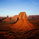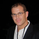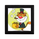8182 vues
Simple Code for Hourly Pivot Points Requested by user pippo

Inputs Tab:
Turn On/Off Pivots
Turn On/Off R3/S3
```//created by ChrisMoody by request for pippo
//Hourly Pivots
study(title="CM_Hourly_Pivots", shorttitle="CM_Hourly_Pivots", overlay=true)
sh = input(true, title="Show Hourly Pivots?")
sh3 = input(false, title="Show R3 & S3?")

//Classic Pivot Calculations
pivot = (high + low + close ) / 3.0
r1 = pivot + (pivot - low)
s1 = pivot - (high - pivot)
r2 = pivot + (high - low)
s2 = pivot - (high - low)
r3 = sh3 and r1 + (high - low) ? r1 + (high - low) : na
s3 = sh3 and s1 - (high - low) ? s1 - (high - low) : na

//Daily Pivots
htime_pivot = security(tickerid, '60', pivot)
htime_r1 = security(tickerid, '60', r1)
htime_s1 = security(tickerid, '60', s1)
htime_r2 = security(tickerid, '60', r2)
htime_s2 = security(tickerid, '60', s2)
htime_r3 = security(tickerid, '60', r3)
htime_s3 = security(tickerid, '60', s3)

plot(sh and htime_pivot ? htime_pivot : na, title="Hourly Pivot",style=circles, color=fuchsia,linewidth=3)
plot(sh and htime_r1 ? htime_r1 : na, title="Hourly R1",style=circles, color=#DC143C,linewidth=3)
plot(sh and htime_s1 ? htime_s1 : na, title="Hourly S1",style=circles, color=lime,linewidth=3)
plot(sh and htime_r2 ? htime_r2 : na, title="Hourly R2",style=circles, color=maroon,linewidth=3)
plot(sh and htime_s2 ? htime_s2 : na, title="Hourly S2",style=circles, color=#228B22,linewidth=3)
plot(sh and htime_r3 ? htime_r3 : na, title="Hourly R3",style=circles, color=#FA8072,linewidth=3)
plot(sh and htime_s3 ? htime_s3 : na, title="Hourly S3",style=circles, color=#CD5C5C,linewidth=3)```

## CommentairesDear Chris, thank you very much indeed for the hourly pivot points. It took you soo little time to make it. Have you a code for Elliott Wave counts, as I choice the points on the chart and it gives the count?
Kindest regards.
Répondrepippo
It actually took me less than two minutes....I had the formula for Daily and above...I only had to change one word...So it was no problem at all.
RépondreWas it the same for the daily that you publisher recently?
Répondre2use
I've published one that does Daily, Weekly, Monthly, Quarterly, Yearly. I tried to add the hourly to that script but the script was too long...So I had to Create a new Version...
Répondre